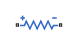# SPICE Resistor

Model SPICE-compatible resistor

•Libraries:
Simscape / Electrical / Additional Components / SPICE Passives

## Description

The SPICE Resistor block represents a SPICE-compatible resistor. You can specify the resistance in one of the following ways:

• As a resistance value

• As process information that the block uses to calculate a resistance value

The block models temperature dependence. There are two ways to specify the resistor temperature:

• When you select `Device temperature` for the Model temperature dependence using parameter, the resistor temperature is

`$T={T}_{C}+{T}_{O}$`

where:

• TC is the Temperature parameter value from the Environment Parameters block. If this block doesn't exist in the circuit, TC is the default value of this parameter.

• TO is the Offset local circuit temperature, TOFFSET parameter value.

• When you select `Fixed temperature` for the Model temperature dependence using parameter, the resistor temperature is the Fixed circuit temperature, TFIXED parameter value.

The block adjusts the specified or calculated resistance value for temperature using the following equation:

R = R0(1+TC1(TTnom)+TC2(TTnom)2)

Where

• R0 is the specified or calculated resistance value.

• TC1 is the First order temperature coefficient, TC1 parameter value.

• TC2 is the Second order temperature coefficient, TC2 parameter value.

• Tnom is the Parameter extraction temperature, TMEAS parameter value.

## Ports

### Conserving

expand all

Electrical conserving port associated with the SPICE resistor positive voltage terminal

Electrical conserving port associated with the SPICE resistor negative voltage terminal

## Parameters

expand all

### Resistance

Number of parallel resistors that the block represents. This value multiplies the output current.

Select one of the following options for specifying the resistor value:

• `Use specified resistance` — Provide the resistance value directly.

• ```Calculate from process information``` — Provide process parameters that the block uses to calculate the resistance value.

When you select this option, the block calculates the resistance using the following equation:

`$R=RSH*\frac{\left(LENGTH-NARROW\right)}{\left(WIDTH-NARROW\right)}$`

where:

• RSH is the Sheet resistance, RSH parameter value.

• LENGTH is the Resistor length, LENGTH parameter value.

• WIDTH is the Resistor width, WIDTH parameter value.

• NARROW is the Etch narrowing, NARROW parameter value.

Resistance value.

#### Dependencies

This parameter is visible only when you select ```Use specified resistance``` for the Resistor parameterization parameter.

Resistance per square of the resistor.

#### Dependencies

This parameter is visible only when you select `Calculate from process information` for the Resistor parameterization parameter.

Length dimension of the resistor.

#### Dependencies

This parameter is visible only when you select `Calculate from process information` for the Resistor parameterization parameter.

Width dimension of the resistor.

#### Dependencies

This parameter is visible only when you select `Calculate from process information` for the Resistor parameterization parameter.

Amount by which the resistor length and width are reduced due to side etching.

#### Dependencies

This parameter is visible only when you select `Calculate from process information` for the Resistor parameterization parameter.

### Temperature

Select one of the following options for modeling the resistor temperature dependence:

• `Device temperature` — Use the device temperature, which is the Temperature parameter value (from the Environment Parameters block, if one exists in the circuit, or the default value for this block otherwise) plus the Offset local circuit temperature, TOFFSET parameter value.

• `Fixed temperature` — Use a temperature that is independent of the circuit temperature to model temperature dependence.

Coefficient for the linear term in the equation that the block uses to adjust the specified or calculated resistance value for temperature.

Coefficient for the quadratic term in the equation the block uses to adjust the specified or calculated resistance value for temperature.

The amount by which the resistor temperature differs from the circuit temperature.

#### Dependencies

This parameter is visible only when you select ```Device temperature``` for the Model temperature dependence using parameter.

The temperature at which to simulate the resistor.

#### Dependencies

This parameter is visible only when you select ```Fixed temperature``` for the Model temperature dependence using parameter.

The temperature at which the resistor parameters were measured.

## Version History

Introduced in R2012b